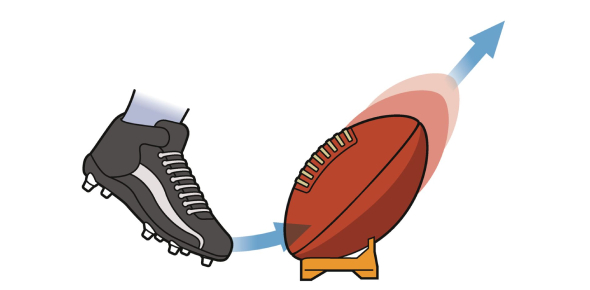# Force And Motion Quiz Test!

15 Questions | Total Attempts: 66Settings.

• 1.
Which must happen for a moving car to change direction?
• A.

The car must slow down.

• B.

Gravity must act on the car.

• C.

The mass of the car must change.

• D.

Another force must act on the car.

• 2.
A train travels 100 meters in 10 seconds. It travels 300 meters in the next 10 seconds. By 30 seconds, it has traveled 900 meters. Which best describes the motion of the train?
• A.

Its speed is decreasing.

• B.

Its speed is increasing.

• C.

Its speed cannot be measured.

• D.

Its speed is remaining constant.

• 3.
What is necessary to determine the speed of an object?
• A.

The mass and volume of the object

• B.

The shape of the object and the distance it moved

• C.

the mass of the object and the time it took to move

• D.

The distance moved by the object and the time it took to move

• 4.
Two cars, a heavier car, and a lighter car are pushed with equal force, and are affected by the same amount of friction. Which best describes the effect on the distance the cars will move?
• A.

The lighter car will travel a longer distance because it has more mass.

• B.

The heavier car will travel a shorter distance because it has more mass.

• C.

The lighter car will travel a shorter distance because its wheels will roll more quickly.

• D.

The heavier car will travel a longer distance because its wheels will roll more slowly.

• 5.
Jim played ice hockey with his friends. When he hit the puck, it stopped before reaching the goal. Which most likely caused this to happen?
• A.

mass

• B.

friction

• C.

Gravity

• D.

Momentum

• 6.
John stands at the top of a steep hill and pushes a ball over the edge with his foot. Which force causes the ball to continue rolling down the hill?
• A.

Mass

• B.

Gravity

• C.

friction

• D.

air resistance

• 7.
Jack and Trish pulled opposite ends of a rope. Neither person was able to move the rope. What can be said about the force used by each person?
• A.

Jack and Trish used no force.

• B.

Jack and Trish used equal force.

• C.

Jack exerted less force than Trish.

• D.

Jack exerted more force than Trish.

• 8.
Which best describes the relationship between movement and friction?
• A.

As the rate of friction increases, the rate of movement increases.

• B.

As the rate of friction increases, the rate of movement decreases.

• C.

As the rate of friction decreases, the rate of movement decreases.

• D.

As the rate of friction decreases, the rate of movement is unchanged.

• 9.
Two dogs are pulling on a toy in opposite directions. Which could cause the toy to move in a specific direction?
• A.

if there is no friction acting on the toy

• B.

If there is no gravity acting on the toy

• C.

if the dogs keep pulling with the same amount of force

• D.

if one dog begins pulling with a greater amount of force

• 10.
A train travels 150 miles in 2 hours. What is the average speed of the train? (average speed = distance ÷ time)
• A.

30 miles per hour

• B.

50 miles per hour

• C.

75 miles per hour

• D.

150 miles per hour

• 11.
If a small rock and a large rock are both thrown at a wall at exactly the same speed, which rock would most likely cause more damage to the wall?
• A.

The large rock would cause more damage because the force would be spread out on a larger area.

• B.

The large rock would cause more damage because it has a greater mass, making the momentum greater.

• C.

The small rock would cause more damage because it has a smaller mass, making the momentum greater.

• D.

The small rock would cause more damage because all of the force would be concentrated on one small area.

• 12.
A car is moving at a constant speed. What is the speed of the car if it moves 100 miles in 2 hours? (speed = distance ÷ time)
• A.

25 miles per hour

• B.

50 miles per hour

• C.

75 miles per hour

• D.

100 miles per hour

• 13.
An airplane traveled 400 miles in one hour. By the end of the next hour, it had traveled a total of 800 miles. Which best describes the motion of the airplane?
• A.

It was moving at a faster speed during the first hour.

• B.

It was moving at a faster speed during the second hour.

• C.

It was moving at the same average speed for the two hours.

• D.

The airplane stopped moving between the first and second hours.

• 14.
For how long did he walk when he started walking the second time?
• A.

2 seconds

• B.

4 seconds

• C.

6 seconds

• D.

8 seconds

• 15.
At what time did the helicopter go back to its original position?
• A.

2 minutes

• B.

4 minutes

• C.

6 minutes

• D.

8 minutes

Related TopicsBack to top